# Experimental Investigation of Thermal Performance Parameters of Drying Air Unit with a Different Flowrate of Liquid Desiccant Solution

Experimental Investigation of Thermal Performance Parameters of Drying Air Unit with a Different Flowrate of Liquid Desiccant Solution
Department of Mechanical Engineering, College of Engineering, Wasit University, Wasit 52001, Iraq

Corresponding Author Email:
mgalazawy@uowasit.edu.iq
Page:
815-820
|
DOI:
https://doi.org/10.18280/ijht.400321
1 May 2022
|
Accepted:
26 May 2022
|
Published:
30 June 2022
| Citation

OPEN ACCESS

Abstract:

Liquid desiccant air-conditioning systems remove directly the latent load from moist air and remove indirectly sensible load of this air by using an indirect evaporative cooling process. The liquid desiccant must subsequently be regeneration once again with a low-grade heat source like solar energy. In this study, calcium chloride solution was used as a desiccant. For the desiccant solution regeneration process, a flat plate solar collector was employed. Different variables, such as the primary airflow rate and desiccant flow rate, were changed during the experiments. The impact of these variables on the performance parameters of the desiccant systems such as moisture removal rate, moisture efficiency, enthalpy efficiency, sensible heat ratio, and the mass transfer coefficient was studied. The obtained results revealed that as the flow rate of primary air increases, the moisture removal rate, sensible heat ratio, and the mass transfer coefficient increase. For example, from 0.006 m/s to 0.0125m/s when the mass airflow rate increased from 0.1 kg/s to 0.18 kg/s.

Keywords:

liquid desiccant solution, air-conditioning systems, dehumidification devices, calcium chloride solution

1. Introduction

The air conditioning industry has become more interested in desiccant dehumidification devices in recent years . Statistics show that an individual's performance in a conditioned space is superior to that in an untreated setting . Moreover, there has been a resurgence of interest in solar-powered air conditioning . Because the cooling load is closely connected to overall solar availability, solar cooling is a viable use of solar thermal technology . Desiccant dehumidification is a technology that has long been used in both industrial and agricultural applications, such as humidity management in textile mills and post-harvest crop drying. In stores, they are drying. In the last few years, all cooling coils including (DX) and chilled-water coils are ineffective moisture removes . Desiccants, on the other hand, can be useful tools and help maintain a pleasant and healthy indoor environment . When compared to traditional compression refrigeration devices, these systems offer the potential to minimize energy usage for humidity management by using low-temperature waste heat . Furthermore, both sensible and latent cooling loads can be separately regulated . The rising demand for air conditioning has resulted in a huge increase in energy resource consumption. One of the ecologically friendly approaches that might assist solve the problem is solar powered cooling [9, 10]. The climate has a big role in deciding which type of evaporative cooler to use . The partial water vapor pressure of the air rises as the air inlet humidity ratio rises, increasing the mass transfer potential, according to Moon et al. . Furthermore, Ahmed and Abdalla in 2012  proved that the moisture removal rate was enhanced by increasing the inlet air flow rate and the inlet air humidity ratio. In the work of Ahmed and Kamal (2012)  the rate of moisture removal was tested in an experiment during which the entry parameters such as: rate, humidity ratio, desiccant flow rate, and concentration are changed. Bouzenada et al. (2015)  investigated experimentally the effectiveness of a low-flow falling-film liquid desiccant air-conditioner. The dehumidifier is one of the most important components in open-cycle liquid desiccant air-conditioning systems. Zouaoui et al. (2016) used packed bed of silica gel particles in order to investigate the air dehumidification and regeneration operations experimentally during adsorption and desorption phases . Rafique et al. (2017)  presented an experimental tests to enhanced liquid desiccant evaporative cooling system. The impact of weather and other factors on device efficiency have been investigated. Ou et al. (2018)  studied the heat and mass transfer properties of a liquid desiccant cooling and dehumidification system in an experimental setting (LDCD). The heat efficiency and moisture efficiency improve as the chilled water flow rate, desiccant solvent flow rate, and concentration increase. Recently, Ebrahimpour et al. used lithium chloride as fluid in liquid desiccant air conditioners . Previously, most published research focused on utilizing an expensive desiccant to remove moisture from air in a basic system such as a pipe or duct [19, 20]. In the current study, the authors combined an indirect evaporative cooling system with a calcium chloride desiccant, which is the cheapest and most readily available desiccant for modern air conditioning applications. In this work, to exchange moisture between the air and the liquid desiccant, the scientists used an experimental counter-flow enthalpy exchanger. The ambient air moisture content reduced by roughly 20% during the dehumidification process and increased by around 14.28 percent during the regeneration phase, according to the findings.

The main aim of this research is to study the effect of the primary air flow rate and desiccant flow rate on desiccant system parameters such as: the moisture removal, efficiency of moisture, enthalpy efficiency, sensible heat ratio and mass transfer coefficient. For this the experiment test rig shown Figure 1 was used. According to that, the main contribution is using calcium chloride as a desiccant solution in the air conditioning system of the present work which includes two air passages one for the primary air and the second for secondary air and joined by cross flow heat and mass exchanger and for the desiccant solution regeneration process, a flat plate solar collector was employed.

2. Description of Experimental Apparatus

The test rig that used in the present investigation consists of the following parts as shown in Figure 1:

• Cross-flow plate heat exchanger.
• Air system inlet Centrifugal fan of the primary and secondary air streams, Air heater, Steam generator (humidifier), water distributor in secondary air streams for Evaporative cooling and air flow regulator.
• Liquid desiccant system includes desiccant distributor in primary air stream, Liquid desiccant storage tank and desiccant Pump.
• Solar Collector for regeneration process of Liquid desiccant.
• Measuring devices includes, temperature and humidity relative measuring devices, Arduino device, water and desiccant solution flow meters, digital thermometer, velocity measuring device (thermo anemometer).

The heat exchanger is essentially made up of thin aluminum sheets that are organized in a precise way to allow two perpendicular air streams (primary and secondary) to flow between parallel planes in alternate passageways. The heat exchanger construction is made up of fifty-two aluminum plates with a thickness of 0.5mm and a 5mm gap between them. Each air stream can flow through 26 channels to the heat exchanger's aluminum plate layout. Steel wire mesh of 0.5 mm square corrugations is placed between the plates to provide turbulence and enhance heat and mass transfer. Figure 2 shows the cross-flow plate heat exchanger.

## 1.png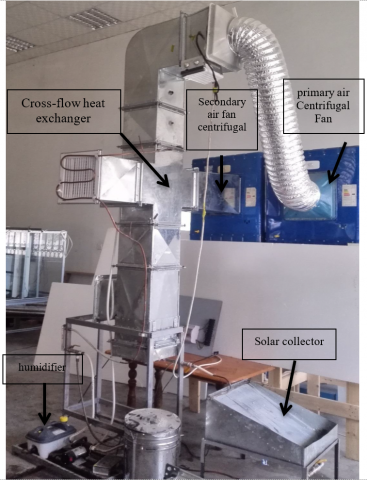(a) Snapshot of experimental teat rig

## 1-2.png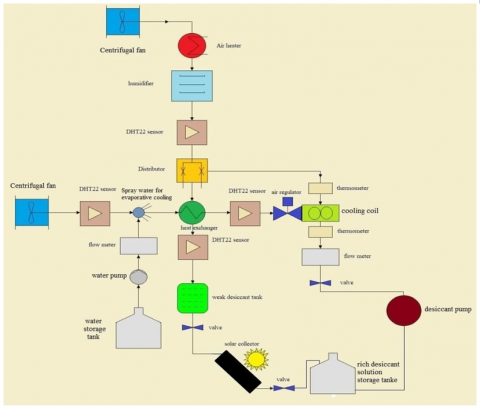(b) Schematic diagram of experiment test rig

Figure 1. The experimental rig

## 2.png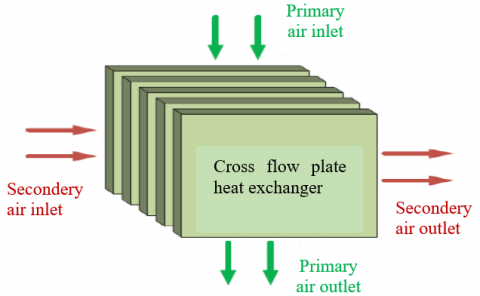Figure 2. Cross-flow plate heat exchanger

3. Performance Parameter of the Heat Exchanger

The performance of desiccant system can be evaluated by calculation the moisture removal rate (MR) from Eq. (1) , the rate of moisture removal is :

$M R=\dot{m}_{a}\left(W_{i-} W_{o}\right)$       (1)

where, $\dot{m}_{a}$ the mass flow is rate of air with unit of Kg/s and (Wi-Wo) is the difference between the moisture content at inlet and outlet with units of Kgv/Kgdryair, respectively. According to the concept of efficiency, the performance of process of removing moisture from the air in the desiccant drter system can be expressed in terms of both the moisture efficiency and the enthalpy efficiency, which can be calculated, respectively, from Eqns. (2) and (3) as follows .

$\eta_{m}=\frac{\left(W_{i-} W_{o}\right)}{\left(W_{i-} W_{e}\right)}$      (2)

$\eta_{h}=\frac{\left(h_{i-} h_{o}\right)}{\left(h_{i-} h_{e}\right)}$      (3)

The sensible heat ratio which is defined as the ratio between the sensible heat load and the total heat load of the air represents the needed handling capability of the air conditioner's various components.

Therefore, Calculating Sensible Heat Ratio (SHR) based on inlet and output air parameters might be used to assess cooling and dehumidify introduction the humidity and temperature, it can be calculated as shown in Eq. (4) :

$S H R=\frac{C_{a}\left(t_{i-} t_{o}\right)}{\left(h_{i-} h_{o}\right)}$      (4)

The Antoine equation is one of the most widely used formulas for determining the vapor pressure of desiccant solution. It can commonly be written as follows :

$\mathrm{p}_{s o l}=a \exp \left(A-\frac{\mathrm{B}}{\mathrm{T}+\mathrm{C}}\right)$      (5)

where, A, B, and C are constants depending on the liquid desiccant temperature and concentration of the desiccant solution.

$a=a_{0}+a_{1} x_{m}+a_{2} x_{m}^{2}+a_{3} x_{m}^{3}+a_{4} x_{m}^{4}$      (6)

in which xm represents the concertation of the solution. Regarding calcium chloride solution used as a liquid desiccant in the Antoine equation for listed in the following Table 1:

Table 1. Antoine’s equation constant for calcium chloride solution

 a0 a1 a2 a3 a4 A B C 0.9899 -8.67 E-3 -3.68 E-2 4.624E-3 -1.67 E-4 18.3036 3816.44 46.13

The equilibrium humidity ratio (We) of the moisture air in contact with calcium chloride solution can be written for an ideal gas as follows Eq. (7):

$W_{e}=0.626185\left(\frac{p_{\text {sol }}}{p_{a t m}+p_{s o l}}\right)$      (7)

In term of equilibrium humidity ratio, the following equation is used to compute the coefficient of mass transfer between calcium chloride solution and the processed air.

$K_{d}=\frac{m_{a}\left(w_{i-} w_{o}\right)}{A \rho\left(w_{i-} w_{e}\right)}$      (8)

4. Results and Discussion

4.1 Mass air flow rate effect

The influence of the process air flow rate on the moisture removal rate is shown in Figure 3. It's worth noting that the moisture removal rate rises as the air flow rate rises. Increasing the air flow rate will remove dehumidified air move quickly for from the interface area thus reducing the moisture gradient between the moist air stream and the desiccant solution. Thus an improvement in the mass transfer coefficient can occur, resulting in an increase in the rate of moisture removed. An increase in the air flow rate can also have a negative effect of decreasing the contact time between the moist and the liquid desiccant.

Figures 4 and 5 represent the effect of the air flow rate on moisture efficiency and enthalpy efficiency, it is noticed from these figures that these two efficiencies decrease with the increase in the air flow rate. The reason for this can be attributed to the fact that the increase in the air flow rate reduces both the moisture content difference and the heat content difference on both sides of the heat exchanger, $\left(w_{i-} w_{o}\right),\left(h_{i-} h_{o}\right)$, respectively. Thus, when referring to Eqns. (2) and (3), this will load to a decrease in the value of these two efficiencies with an increase in the air flow rate.

## 3.png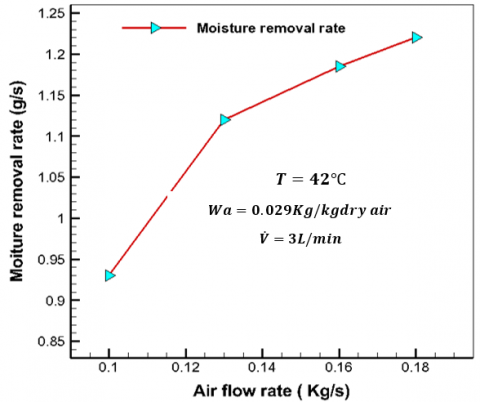Figure 3. Effect of air flow rate on moisture removal

## 4.png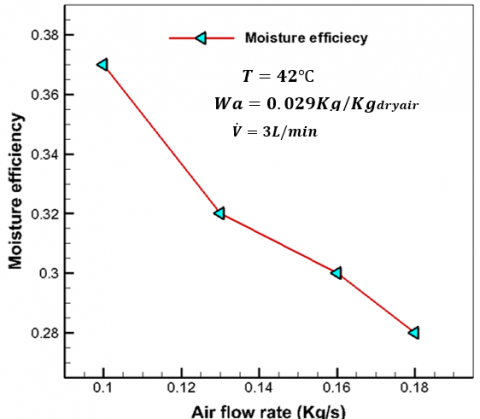Figure 4. Effect of air flow rate on moisture efficiency

## 5.png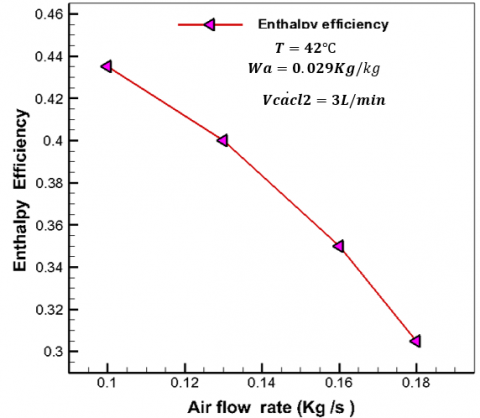Figure 5. Effect of air flow rate on enthalpy efficiency

## 6.png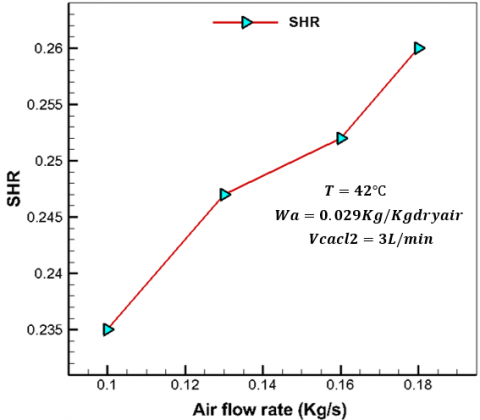Figure 6. Effect of air flow rate on (SHR)

## 7.png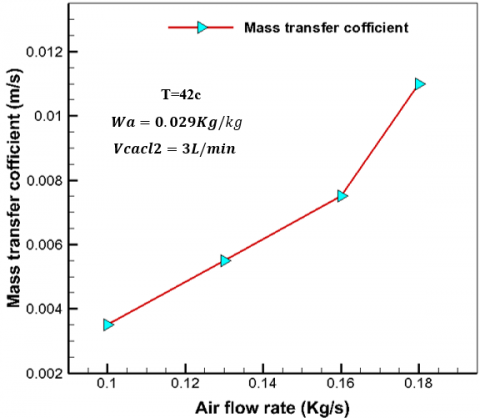Figure 7. Effect of airflow rate on the mass transfer coefficient

Figure 6 shows, in general, an increase in the values of the sensible heat ratio (which represent the ratio between the sensible heat load to the total heat removed from the process air through the heat exchanger) with the increase in the air flow rate. This could be due to the fact that the increase in the air flow rate will make a difference in the air temperature on both side of the heat exchanger more than the differences in enthalpy. Returning to Eq. (4), this means that there will be an increasing in the sensible heat ratio (SHR) with the increase in the air flow rate.

Figure 7 shows the change in mass transfer coefficient values with change in the air flow rate. An increase in the air flow rate loads to an increase in the mass transfer coefficient. The reason for this can be explained by the fact that an increase in the air flow rate means an increase in its velocity on the surfaces of heat and mass exchanger through the heat exchanger, which leads to a decrease in the thermal resistance to heat and mass transfer on these surface.

4.2 Liquid desiccant flow rate effect

The effect of the desiccant solution flow rate on the moisture removal rate is shown in Figure 8. As is clear in the figure, an increase in the flow rate of the desiccant solution lead to an increase in the rates of moisture removal. This is due to the good wetting of the surfaces of the cross-flow heat exchanger resulting from the use of high flow rate of the desiccant solution.

Figures 9 and 10 shown respectively, the effect of a change in solution leads to increase in both moisture efficiency and enthalpy efficiency. This can be explained by referring to Eqns. (3) and (4), the increase in the in the flow rate of the flow desiccant solution leads to a decreases difference in the values of moisture content and enthalpy of moist air on both side of the heat exchanger. Thus the values of these efficiencies will increase with the increases in the flow rate of the solution.

## 8.png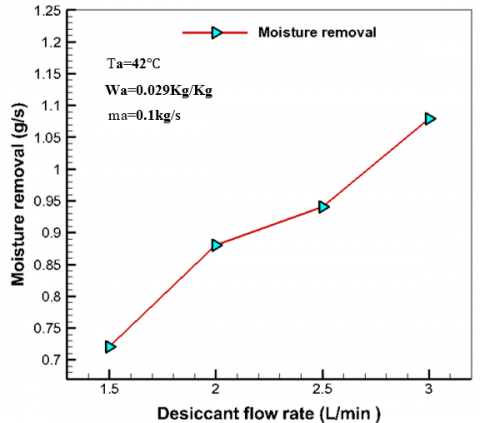Figure 8. Effect of desiccant flow rate on moisture removal

## 9.png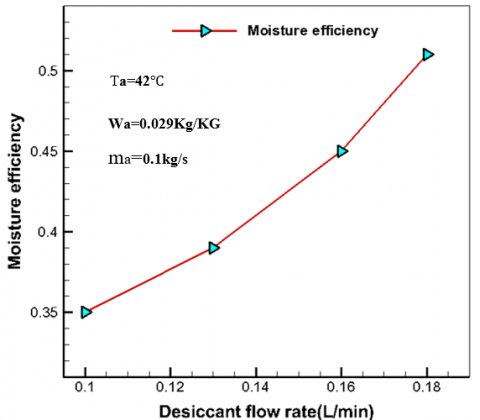Figure 9. Effect of desiccant flow rate on moisture efficiency

## 10.png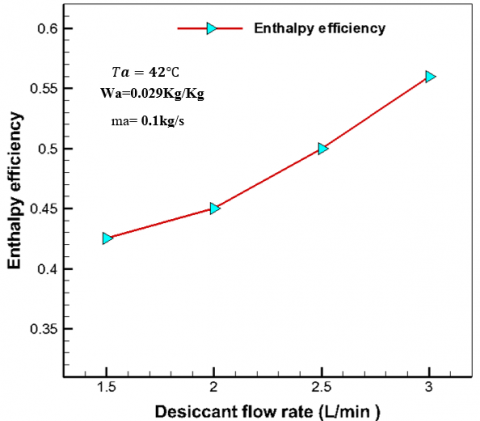Figure 10. Influence of desiccant flow rate on enthalpy efficiency

Figure 11 describes the effect of a change in desiccant solution floe rates on the SHR values. As is clear from the figure, increasing the flow rate of the desiccant solution leads to a decrease in the SHR.

## 11.png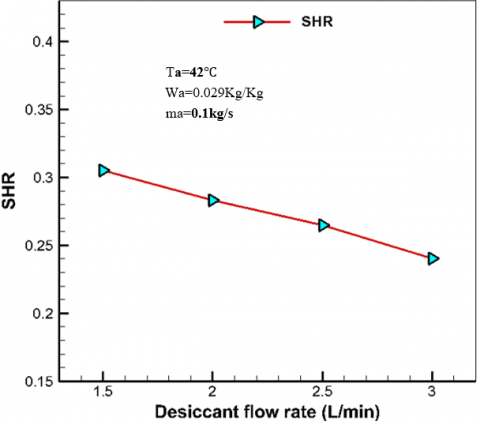Figure 11. Effect of desiccant flow rate on (SHR)

## 12.png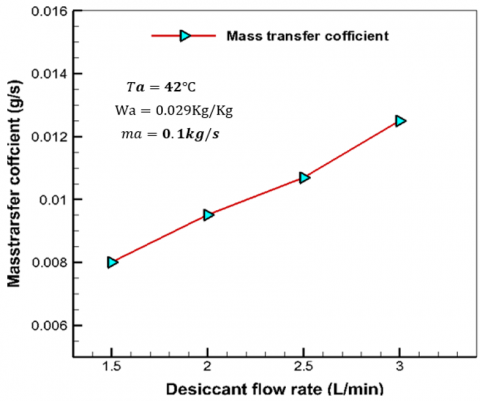Figure 12. Behaviour of desiccant flow rate with mass transfer coefficient

The reason for this can be attributed to the fact that increasing the rate of flow of the solution leads to a difference in the heat content of the moist air on both side of the heat exchanger more flow the difference in the temperature of this air therefore, when referring to equation, it can be concluded that increase in the flow rate of the desiccant solution leads to a decrease in the values of the SHR.

Th influence of the solution flow rate on the mass transfer coefficient is seen in Figure 12. An increase in the desiccant solution flow rate leads, in general, to an improvement in the values of the mass transfer coefficient. It can also be seen from the figure that increasing the flow rate of the solution from 1.5 L/min to 3 L/min loads to an increase rate in the mass transfer coefficient values less that the increaser ate are lower. therefore, increasing the flow rate of the desiccant more than 3 L/min, it will be difficult to expect its contribution to improving the mass transfer coefficient.

5. Conclusions

From the results obtained from this study, the following conclusions can be drawn:

(1) The moisture removal rate increases with the increase of process air flow rate and desiccant solution flow rate.

(2) Moisture efficiency and enthalpy efficiency decrease with increase process air flow rate which these efficiency increase with the increase in the flow rate of the desiccant solution.

(3) The SHR increase with the increase in the air flow rate and decrease with the increase in the desiccant solution flow rate.

(4) An increase in both the air flow rate and the desiccant solution flow rate leads to an increase in the mass transfer coefficient values.

References

 Ramli, M.S.A., Misha, S., Haminudin, N.F., Rosli, M.A.M., Yusof, A.A., Md Basar, M.F., Sopian, K., Ibrahim, A., Abdullah, A.F. (2021). Review of desiccant in the drying and air-conditioning application. International Journal of Heat and Technology, 39(5): 1475-1482. https://doi.org/10.18280/ijht.390509

 Rafique, M.M., Gandhidasan, P., Rehman, S., Al-Hadhrami, L.M. (2015). A review on desiccant based evaporative cooling systems. Renewable and Sustainable Energy Reviews, 45: 145-159. https://doi.org/10.1016/j.rser.2015.01.051

 Mesquita, L.C.S., Harrison, S.J., Thomey, D. (2006). Modeling of heat and mass transfer in parallel plate liquid-desiccant dehumidifiers. Solar Energy, 80(11): 1475-1482. https://doi.org/10.1016/j.solener.2006.03.003

 Crofoot, L., Harrison, S. (2012). Performance evaluation of a liquid desiccant solar air conditioning system. Energy Procedia, 30: 542-550. https://doi.org/10.1016/j.egypro.2012.11.064

 Jradi, M., Riffat, S. (2014). Energy performance of an innovative liquid desiccant dehumidification system with a counter-flow heat and mass exchanger using potassium formate. Renewable Bioresources, 2(1): 5. https://doi.org/10.7243/2052-6237-2-5

 Elhelw, M. (2016). Performance evaluation for solar liquid desiccant air dehumidification system. Alexandria Engineering Journal, 55(2): 933-940. https://doi.org/10.1016/j.aej.2016.02.021

 Al-Abboodi, N.K.F., Khalaf, K.A., Ridha, H., Al-Azawy, M.G. (2022). Thermal and flow analysis of different shaped pin fins for improved heat transfer rate. International Journal of Heat and Technology, 40(1): 201-210. https://doi.org/10.18280/ijht.400124

 Kubota, M., Hanada, T., Yabe, S., Matsuda, H. (2013). Regeneration characteristics of desiccant rotor with microwave and hot-air heating. Applied Thermal Engineering, 50(2): 1576-1581. https://doi.org/10.1016/j.applthermaleng.2011.11.044

 Bouzenada, S., Salmon, T., Fraikin, L., Kaabi, A., Léonard, A. (2014). Experimental study on mass transfer comparison of two liquid desiccants aqueous solutions. In International Conference on Renewable Energies and Power Quality (ICREPQ’14), Cordoba (Spain), pp. 665-670. https://doi.org/10.24084/repqj12.446

 Assari, M.R., Mirzavand, R., Basirat Tabrizi, H., Jafar Gholi Beik, A.R. (2022). Effect of steps height and glass cover angle on heat transfer performance for solar distillation: Numerical study. International Journal of Engineering, 35(1): 237-247. https://doi.org/10.5829/IJE.2022.35.01A.23

 Moon, C.G., Bansal, P.K., Jain, S. (2009). New mass transfer performance data of a cross-flow liquid desiccant dehumidification system. International Journal of Refrigeration, 32(3): 524-533. https://doi.org/10.1016/j.ijrefrig.2008.06.011

 Ahmed, A.M., Abdalla, K.N. (2012). Experimental study of heat and mass transfer of Solar Powered liquid desiccant regeneration system. Proceedings, 1: 299-304.

 Ahmed, A.M., Abdalla, K.N. (2012). Moisture removal rate in a solar powered liquid desiccant air conditioning system. University Of Khartoum Engineering Journal, pp. 344-352.

 Bouzenada, S., McNevin, C., Harrison, S., Kaabi, A. N. (2015). An experimental study on the dehumidification performance of a low-flow falling-film liquid desiccant air-conditioner. Procedia Computer Science, 52: 796-803. https://doi.org/10.1016/j.procs.2015.05.135

 Zouaoui, A., Zili-Ghedira, L., Nasrallah, S.B. (2016). Experimental investigation of air dehumidification and regeneration operations using packed bed of silica gel particles. International Journal of Heat and Technology, 34(1): 103-109. https://doi.org/10.18280/ijht.340115

 Rafique, M.M., Rehman, S., Alhems, L.M., Ali Shakir, M. (2017). A liquid desiccant enhanced two stage evaporative cooling system—Development and performance evaluation of a test rig. Energies, 11(1): 72. https://doi.org/10.3390/en11010072

 Ou, X., Cai, W., He, X., Zhai, D. (2018). Experimental investigations on heat and mass transfer performances of a liquid desiccant cooling and dehumidification system. Applied Energy, 220: 164-175. https://doi.org/10.1016/j.apenergy.2018.03.087

 Ebrahimpour, B., Goudarzi, A.M., Kaviani, A. (2022). Enhancing performance of an air conditioner by preheating and precooling of liquid desiccant and non-processed air. International Journal of Engineering, 35(2): 425-432. https://doi.org/10.5829/ije.2022.35.02b.19

 Ridha, H., Al-Azawy, M.G. (2021). Effect of wall heat transfer on the fluidization process. International Journal of Heat and Technology, 39(2): 615-620. https://doi.org/10.18280/ijht.390232

 Ridha, H., Al-Azawy, M.G. (2021). Study of the effect of particle diameters on two-phase (air-solid) fluidization bed hydrodynamics. Materials Today: Proceedings, 42: 2113-2118. https://doi.org/10.1016/j.matpr.2020.12.294

 Murathathunyaluk, S., Kitchaiya, P. (2021). Theoretical study on a novel temperature breakpoint cyclic operation to enhance desiccant packed bed performance. International Journal of Heat and Technology, 39(4): 1134-1142. https://doi.org/10.18280/ijht.390411

 Elsarrag, E. (2007). Moisture removal rate for air dehumidification by triethylene glycol in a structured packed column. Energy conversion and management, 48(1): 327-332. https://doi.org/10.1016/j.enconman.2006.04.013

 Gao, W.Z., Cheng, Y.P., Jiang, A.G., Liu, T., Anderson, K. (2015). Experimental investigation on integrated liquid desiccant–Indirect evaporative air cooling system utilizing the Maisotesenko–Cycle. Applied Thermal Engineering, 88: 288-296. https://doi.org/10.1016/j.applthermaleng.2014.08.066

 Saman, W.Y., Alizadeh, S. (2002). An experimental study of a cross-flow type plate heat exchanger for dehumidification/cooling. Solar Energy, 73(1): 59-71. https://doi.org/10.1016/S0038-092X(01)00078-0